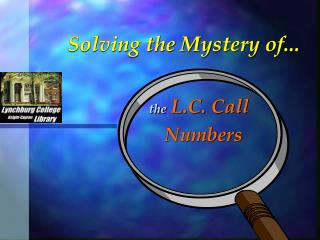DownloadDownload PresentationSolving the Mystery of...

# Solving the Mystery of...

Download Presentation## Solving the Mystery of...

- - - - - - - - - - - - - - - - - - - - - - - - - - - E N D - - - - - - - - - - - - - - - - - - - - - - - - - - -
##### Presentation Transcript

1. Solving the Mystery of... theL.C. Call Numbers

2. Library of Congress Call Numbers • Call numbers are used to group books by their subjects. • Books with similar topics will shelve together. • Books may fit into more than one subject category. • Call numbers are based on the dominate topic.

3. Library of Congress Classification System Outline • A ~ General works. • Encyclopedias and Almanacs • B - P ~ Humanities and the Social Sciences • Q - V ~ Natural Sciences and Technology

4. Letter combinations are used to express subsets of a major heading. • Q = General Science • QA=Mathematics and Computer Science • QB-QE=Physical Sciences • QH-QR=Biological Sciences

5. The call number for this book would look like this: HV is the letter combination for the subdivision of the Social Sciences dealing with Social & Public Welfare, and Social Pathology. 6025 is the number in the HV group that designates General Works on Criminology. If the Library has severalbooks that are general works on criminology, they would have the same beginning call number, HV6025. Of those books, only this one would have a call number that ends in .C582 2000. HV 6025 .C582 2000 This indicates the year the item was published. This letter-number combination indicates the specific title or author’s last name. Decoding a Call Number Consider the book: Introducing Criminology by Clive Coleman

6. Shelving by Call Numbers Books are arranged in alphabetical order by the first letter combination of the call number, then in numerical order by the first set of numbers., then in alphabetical order by the specific book section of the call number. The shelf where the book “Introducing Criminology” is shelved might look like this: HV 6021 .S33 HV 6025 .C582 2000 HV 6025 .C65 HV 6025 .D34 1997 HV 6025 .M6 HV 6025 .R47 HV 6028 .B44

7. Call Number numbers The first number in every call number is a whole number. HV 27 .C76 HV 217 .B56 HV 2017 .A45 HV 2017 .A456 HV 2017 .A52 HV 2017 .A6 These numbers are read as “HV twenty-seven, HV two hundred and seventeen, & HV two thousand and seventeen.” When you read them that way, it is apparent that they are in the correct order numerically.

8. Call Number numbers The second set of numbers in each call number are decimal numbers. HV 27 .C76 HV 217 .B56 HV 2017 .A45 HV 2017 .A456 HV 2017 .A52 HV 2017 .A6 This second part of this number would be read as “point A four hundred fifty-six thousandths” (456/1000). And this number would be “point A fifty two hundredths” (52/100 which is larger that 456/1000 or 45.6/100). So these call numbers are in the correct order numerically.

9. Call Number numbers The second set of numbers in each call number are decimal numbers. HV 27 .C76 HV 217 .B56 HV 2017 .A45 HV 2017 .A456 HV 2017 .A52 HV 2017 .A6 This second part of this number would be read as “point A six tenths”(6/10), which is more than “point A fifty-two hundredths” (52/100 . 6/10 = 60/100). So these call numbers are in the correct order.

10. The End